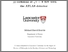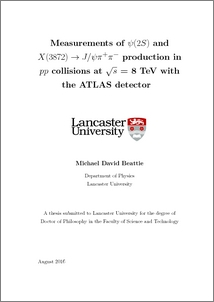# Measurements of ψ(2S) and X(3872) → J/ψ π+π- production in pp collisions at √s = 8 TeV with the ATLAS detector

Beattie, Michael (2016) Measurements of ψ(2S) and X(3872) → J/ψ π+π- production in pp collisions at √s = 8 TeV with the ATLAS detector. PhD thesis, UNSPECIFIED.Preview
PDF (2016beattiephd)
2016beattiephd.pdf - Published Version

## Abstract

Differential cross sections are presented for the prompt and non-prompt production of the hidden-charm states X(3872) and ψ(2S), in the decay mode J/ψπ+π −, measured using 11.4 fb−1 of pp collisions at √ s = 8 TeV by the ATLAS detector at the LHC. The ratio of cross sections X(3872)/ψ(2S) is also given, separately for prompt and non-prompt components, as well as the non-prompt fractions of X(3872) and ψ(2S). Assuming independent single effective lifetimes for non-prompt X(3872) and ψ(2S) production gives RB = Br(B→X(3872) + any)Br(X(3872)→J/ψπ+π−) Br(B→ψ(2S) + any)Br(ψ(2S)→J/ψπ+π−) = (3.95 ± 0.32(stat) ± 0.08(sys))%, while separating short and long-lived contributions, assuming that the short-lived component is due to Bc decays, gives RB = (3.57±0.33(stat)±0.11(sys))%, with the fraction of non-prompt X(3872) produced via Bc decays for pT (X(3872)) > 10 GeV being (25 ± 13(stat) ± 2(sys) ± 5(spin))%. The distributions of the dipion invariant mass in the X(3872) and ψ(2S) decays are also measured and compared to expectations.

Item Type:
Thesis (PhD)
Subjects:
Departments:
ID Code:
84121
Deposited By:
Deposited On:
20 Jan 2017 12:30
Refereed?:
No
Published?:
Published